JEE  >  JEE Advanced Level Test: Vector

# JEE Advanced Level Test: Vector - JEE

Test Description

## 30 Questions MCQ Test Mathematics (Maths) Class 12 - JEE Advanced Level Test: Vector

JEE Advanced Level Test: Vector for JEE 2023 is part of Mathematics (Maths) Class 12 preparation. The JEE Advanced Level Test: Vector questions and answers have been prepared according to the JEE exam syllabus.The JEE Advanced Level Test: Vector MCQs are made for JEE 2023 Exam. Find important definitions, questions, notes, meanings, examples, exercises, MCQs and online tests for JEE Advanced Level Test: Vector below.
Solutions of JEE Advanced Level Test: Vector questions in English are available as part of our Mathematics (Maths) Class 12 for JEE & JEE Advanced Level Test: Vector solutions in Hindi for Mathematics (Maths) Class 12 course. Download more important topics, notes, lectures and mock test series for JEE Exam by signing up for free. Attempt JEE Advanced Level Test: Vector | 30 questions in 60 minutes | Mock test for JEE preparation | Free important questions MCQ to study Mathematics (Maths) Class 12 for JEE Exam | Download free PDF with solutions
 1 Crore+ students have signed up on EduRev. Have you?
JEE Advanced Level Test: Vector - Question 1

### If the vector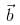is collinear with the vector (2√2, -14) and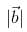= 10, then

Detailed Solution for JEE Advanced Level Test: Vector - Question 1

a = 2(2)½ i -  j + 4k
|b| = 10
[(2(2λ)2 + (1λ)2 + (4λ)2]½
=[ 8λ2 + λ2 + 16λ2]½ = 10
= 25λ2 = (10)2
25λ2 = 100
λ = +-2
b = +-(4(2)½ i - 2j + 8k)
Therefore, 2a +- b

JEE Advanced Level Test: Vector - Question 2

### The vertices of a triangle are A(1, 1, 2), B(4, 3, 1) and C(2, 3, 5). A vector representing the internal bisector of the angle A is

Detailed Solution for JEE Advanced Level Test: Vector - Question 2

Let AD is the bisector of ∠A.Then,
BD/DC = AB/ACeq(1)
Given the vertices of triangle,
AB = √32 + 22 + 12 = √14
AC = √12 + 22 + 32 = √14
As AB = BC, So, BD = DC(from eq(1))
It means D is middle point of BC. So, vertices of D will be (3,3,3).
So, vector AD will be 2iˆ+ 2jˆ+ kˆ.

JEE Advanced Level Test: Vector - Question 3

### Let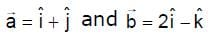. The point of intersection of lines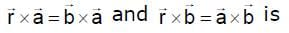JEE Advanced Level Test: Vector - Question 4

If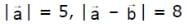and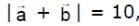then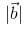is equal to

JEE Advanced Level Test: Vector - Question 5

Angle between diagonals of a parallelogram whose side are represented by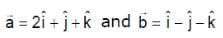Detailed Solution for JEE Advanced Level Test: Vector - Question 5

D1 = a+b
D2 = a-b
D1 = 3i + 0j + 0k
D2 = i + 2j + 2k
|D1| = 3
D1.D2 = |D1| . |D2| . cos θ
3 + 0 + 0 = (3) . (3) cos θ
3 = 9 cosθ
cos-1 = (⅓)

JEE Advanced Level Test: Vector - Question 6

Vector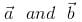make an angle θ = 2π/3. if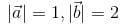, then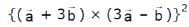is equal to

Detailed Solution for JEE Advanced Level Test: Vector - Question 6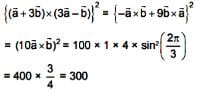JEE Advanced Level Test: Vector - Question 7

Unit vector perpendicular to the plane of the triangle ABC with position vectors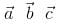of the vertices
A, B, C is

Detailed Solution for JEE Advanced Level Test: Vector - Question 7

Δ = 1/2(a * b) = 1/2(b*c) = 1/2(c*a)
2Δ = a*b = b*c = c*a
unit vector = 1/2Δ[a*b + b*c + c*a]

JEE Advanced Level Test: Vector - Question 8

The value of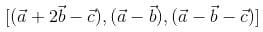is equal to the box product

Detailed Solution for JEE Advanced Level Test: Vector - Question 8

Matrix {(1,2,-1) (1,-1,0) (1,-1,-1)} [a b c]
= {1(1) -2(-1) -1(0)} [a b c]
= 3[a b c]

JEE Advanced Level Test: Vector - Question 9

If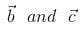are two non-collinear vectors such that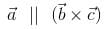, then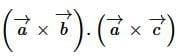is equal to

JEE Advanced Level Test: Vector - Question 10

Vector of length 3 unit which is perpendicular to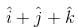and lies in the plane ofand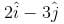Detailed Solution for JEE Advanced Level Test: Vector - Question 10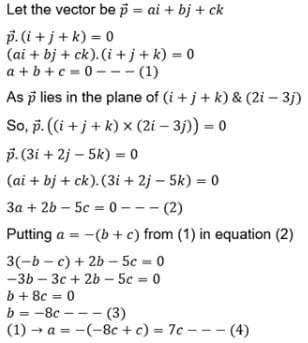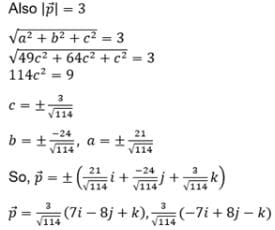JEE Advanced Level Test: Vector - Question 11

Vector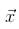satisfying the relation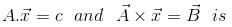Detailed Solution for JEE Advanced Level Test: Vector - Question 11

A * X = B
(A * X)*A = B * A
=> -A(x.A) + x(A.A) = B * A
=> -Ac + x|A|2 = B * A
x|A|2 = B * A + Ac
x = [B * A + Ac]/|A|2

JEE Advanced Level Test: Vector - Question 12

If a ,b,c are linearly independent vectors, then which one of the following set of vectors is linearly dependent ?

Detailed Solution for JEE Advanced Level Test: Vector - Question 12

xa + yb + zc = 0
We have to prove x = y = z = 0
a,b,c are non planner
x(a-b) +y(b-c) +c(c-a) = 0
Let x = 1, y = 1, z = 1
So, we get a - b + b - c + c - a = 0

JEE Advanced Level Test: Vector - Question 13

Let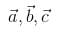be vectors of length 3,4,5 respectively. Let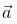be perpendicular to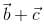,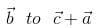and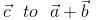. then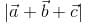Detailed Solution for JEE Advanced Level Test: Vector - Question 13

|A| = 3, |B| = 4, |C| = 5
Since A.(B + C) = B.(C+A) = C(A+B) = 0...........(1)
|A+B+C|2 = |A| + |B|2 + |C|2 + 2(A.B + B+C + C.A)
= 9+16+25+0
from eq(1) {A.B + B+C + C.A = 0}
therefore, |A+B+C|2 = 50
=> |A+B+C| = 5(2)1/2

JEE Advanced Level Test: Vector - Question 14

Given the vertices A (2, 3, 1), B(4, 1, –2), C(6, 3, 7) & D(–5, –4, 8) of a tetrahedron. The length of the altitude drawn from the vertex D is

JEE Advanced Level Test: Vector - Question 15

for a non zero vector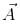If the equations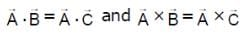hold simultaneously, then

JEE Advanced Level Test: Vector - Question 16

The volume of the parallelopiped constructed on the diagonals of the faces of the given rectangular parallelopiped is m times the volume of the given parallelopiped. Then m is equal to

Detailed Solution for JEE Advanced Level Test: Vector - Question 16

Vi=[a→,b→,c→]
(a→ + b→)(b→ + c→)(a→ + c→)
Vf=[(a→ + b→)(b→ + c→)(a→ + c→)]
=(a→ + b→)⋅[(b→ + c→)⋅(a→ + c→)]
=(a→ +b→)[b→⋅a→ + c→⋅a→ + b→⋅c→ + c→⋅c→]
=[b→ c→ a→]+[a→ b→ c→]
Vf=2[a→ b→ c→]
Vf=2Vi.

JEE Advanced Level Test: Vector - Question 17

If u and v are unit vectors and θ is the acute angle between them, then 2u × 3v is a unit vector for

JEE Advanced Level Test: Vector - Question 18

The value of a, for which the points A,B,C with position vectors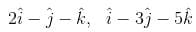and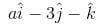respectively are the vertices of a right angled triangle with C = π/2 are

Detailed Solution for JEE Advanced Level Test: Vector - Question 18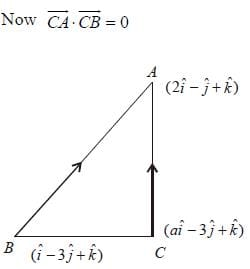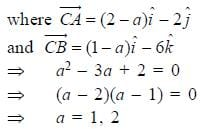JEE Advanced Level Test: Vector - Question 19

The distance between the line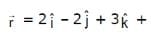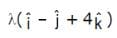and the plane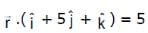is

JEE Advanced Level Test: Vector - Question 20

A particle is acted upon by constant forces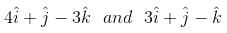which displace ot from a point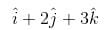to the point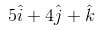. The workdone in standard units by the force is given by

JEE Advanced Level Test: Vector - Question 21

If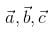are non-coplaner vectors and λ is a real number, then the vectors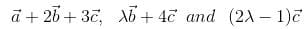are non-coplaner for

JEE Advanced Level Test: Vector - Question 22

Let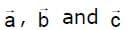be non zero vectors such that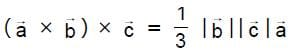, If θ is the acute angle between the vectors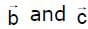, then sin θ equals is

Detailed Solution for JEE Advanced Level Test: Vector - Question 22

(a→*b→)*c→ = (1/3)|b→||c→||a→|
⇒ − c→*(a→*b→) = (1/3)|b→||c→||a→|
⇒(c→.a→)b→ −(c→.b→)a→ =(1/3)|b→||c→|a→|
Now, as all of them are non-collinear, (c→⋅a→) can be 0 that means,
(c→.b→) = (−1/3)|b→||c→|
⇒|b→|.|c→|cosθ = (−1/3)|b→||c→|
⇒ cosθ = −1/3
sinθ = √1−(1/3)^2
= √8/9
= (2√2)/√3

JEE Advanced Level Test: Vector - Question 23are three vectors, such that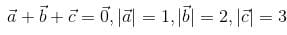then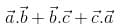is equal to

JEE Advanced Level Test: Vector - Question 24

If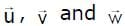are three non-coplaner vectors, then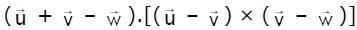equals

JEE Advanced Level Test: Vector - Question 25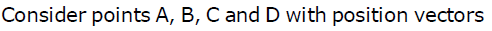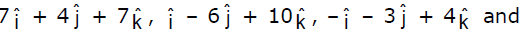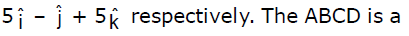JEE Advanced Level Test: Vector - Question 26

The vectors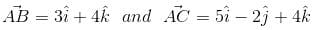are the sides of a triangle ABC. The length of the median through A is

Detailed Solution for JEE Advanced Level Test: Vector - Question 26

The length of median through A = vector(AB + BC)/2
= (3i + 4k + 5i - 2j + 4k)/2
= (8i - 2j + 8k)/2
= 4i - j + 4k
Length  = √(16 + 1 + 16) = √33

JEE Advanced Level Test: Vector - Question 27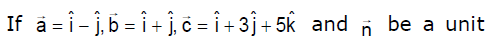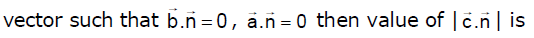JEE Advanced Level Test: Vector - Question 28

If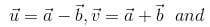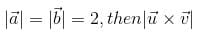is equal to

Detailed Solution for JEE Advanced Level Test: Vector - Question 28

∣u × v∣=∣(a − b) × (a + b)∣
=2∣a × b∣      (∵a × a = b × b = 0)
and ∣a × b∣2 + (a ⋅ b)2
=(ab sinθ)+ (abcosθ)2
=a2b2
⇒∣a×b∣ = a2b2−(a ⋅ b)2
So, ∣u × v∣ = 2∣a × b∣
=2[a ^ 2b− (a ⋅ b)2]1/2
=2[(2)2(2)2−(a⋅b)2]1/2
=2(16−(a⋅b)2)1/2
∴∣a∣=∣b∣=2

JEE Advanced Level Test: Vector - Question 29

Let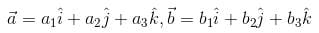and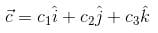be three non-zero vectors such that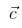is a unit vector perpendicular to both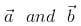. if the angle betweenis π/6, then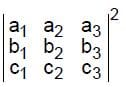is equal to

Detailed Solution for JEE Advanced Level Test: Vector - Question 29

According to the given conditions,
(c1)2+c(2)2+(c3)22 =1,a⋅c=0,b⋅c=0
and cosπ/6 = [(3)1/2]/2
​(a1b1+a2b2+a3b3]/[(a1)2+(a2)2 + (a3)^2)]^1/2 [(b1)^2 + (b2)2 + (b3)2]1/2
Thus a1c1+a2c2+a3c3=0, b1c1+b2c2+b3c3=0
and [(3)^1/2]/2[(a1)2+(a2)2 + (a3)2)1/2 ((b1)2 + (b2)2 +(b3)2]1/2
=a1b1+a2b2 +a3b3
[(a1)2+(a2)2 + (a3)2] -(a1b1 + a2b2 + a3b3)2
[(a1)2+(a2)2 + (a3)2] [(b1)2+(b2)2 + (b3)2]
= -3/4 [(a1)2+(a2)2 + (a3)2] [(b1)2+(b2)2 + (b3)2]
= 1/4 [(a1)2+(a2)2 + (a3)2] [(b1)2+(b2)2 + (b3)2]

JEE Advanced Level Test: Vector - Question 30

A point taken on each median of a triangle divides the median in the ratio 1 : 3, reckoning from the vertex.
Then the ratio of the area of the triangle with vertices at these points to that of the original triangle is

Detailed Solution for JEE Advanced Level Test: Vector - Question 30

Let A (0,0); B (4m , 0) and C(4p , 4q)
M1 (2m + 2p, 2q)
M2 (2p , 2q) and M3 (2m , 0)
Let E , F and G be the point on the median.
E = (2m + 2p) / 4, 2q / 4) = ((m + p) / 2, q / 2)
F = ((2p + 12m) / 4, (2q + 0) / 4) = ((p + 6m) / 2, q / 2)
G = ((2m + 12p) / 4, (0 + 12 q) / 4) = ((m + 6p ) / 2, 3q)
Area of traingle ABC = 1/2
Area of traingle ABC = 1/2 {(0,0,0) (4m,0,1) (4p,4q,1)}
=1/2(16) = 8 unit
Area of triangle EFG = 1/2 {((m + p) / 2, q / 2, 1)) ((p + 6m) / 2, q / 2, 1) ((m + 6p)/2, 3q, 1)}
= 25/8 unit
ar (EFG) /ar (ABC) = {25 / 8} / 8
= 25/64

## Mathematics (Maths) Class 12

206 videos|264 docs|139 tests